# RRB ALP 2018 Practice Test Papers | Arithmetic Questions (Day-68)

Dear Aspirants, Here we have given the Important RRB ALP & Technicians Exam 2018 Practice Test Papers. Candidates those who are preparing for RRB ALP 2018 can practice these Arithmetic Questions to get more confidence to Crack RRB 2018 Examination.

[WpProQuiz 2949]

Click “Start Quiz” to attend these Questions and view Solutions

1) If sin3θ = cos(θ -6°), where 3θ and (θ -6°) are acute angles, then find thevalue of θ.

a) 90°

b) 34°

c) 24°

d) 56°

2).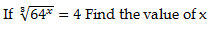a) 1

b) 2

c) 4

d) 6

3) A batsman in his 10th innings makes a Score of 69 and thereby increased his average score by 1. What is his average after the 10thinnings?

a) 50

b) 60

c) 70

d) 55

4) Two persons Contested in an election, Winning person got 450 votes which is 75% of the total vote. How much total vote casted in the election?

a) 720

b) 840

c) 900

d) 600

5) If 7A = 13B = 5C, then A:B:C = ?

a) 65: 35: 91

b) 91: 35: 65

c) 35: 91: 65

d) 91: 65: 35

6) Find the Simple interest of sum Rs.5000 for 2 years at the rate of 20% p.a

a) 2000

b) 4000

c) 7000

d) 5000

7) Amir Purchased a Mobile for 20,000 and sold it for 10% loss. Find the Selling price of the mobile

a) 15000

b) 18000

c) 12000

d) 14000

8) Ages of Kabil, sunil and Deepak are 2: 3: 4 ratio. Which of the following ages can be their correct ages?

a) 14, 15 and 16

b) 30, 17 and 40

c) 5, 15 and 20

d) 10, 15 and 20

9) If 30%(x+y) = 70% (x-y), then Find xy

a) 10

b) 11

c) 15

d) 20

10) A man finished a work in 6 days and B can do the same work in 4 days.  B has left the work after 2 days.In  How many days the work will be completed?

a) 4 days

b) 3 days

c) 2 days

d) 5 days

sin 3θ = cos(θ – 6°)

So, sin3θ = sin {90° – (θ – 6°)}

Or sin3θ = sin (90° – θ + 6°)

Or sin 3θ = sin (96° – θ)

So, 3θ = (96° – θ) [sin B = sin θ means ∠B = θ

Or 3θ + θ = 96°

Or 4θ = 96°

Or θ = 24°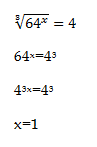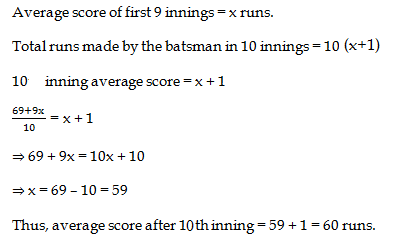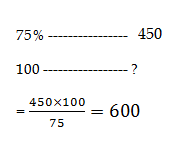)

S.I = (PNR/100) = (5000*2*20)/100 = 2000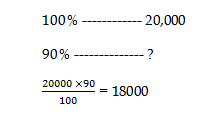10        15        20

2      :      3     :    4

30/100(x+y) = 70/100(x-y)

3(x+y) = 7(x -y)

X+ y =7                       x- y = 3

X = 5; y =2

A – 1/6 days

B – 1/4 days

For 2 days, A + B = 1/6 + 1/4  = (5/12)* 2 = 10/ 12 = 5/6 work complete

Remaining work = 1/ 6 work

(1/6)* 6 = 1 Days

Total days = 2 + 1 = 3 days

RRB ALP 2018 – “All in One” Study Materials and Practice Sets